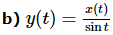Courses

Stable & Unstable Systems Notes | Study Digital Signal Processing - Electrical Engineering (EE)

Electrical Engineering (EE): Stable & Unstable Systems Notes | Study Digital Signal Processing - Electrical Engineering (EE)

The document Stable & Unstable Systems Notes | Study Digital Signal Processing - Electrical Engineering (EE) is a part of the Electrical Engineering (EE) Course Digital Signal Processing.
All you need of Electrical Engineering (EE) at this link: Electrical Engineering (EE)

A stable system satisfies the BIBO (bounded input for bounded output) condition. Here, bounded means finite in amplitude. For a stable system, output should be bounded or finite, for finite or bounded input, at every instant of time.

Some examples of bounded inputs are functions of sine, cosine, DC, signum and unit step.

Examples

a) y(t) = x(t)+10

Here, for a definite bounded input, we can get definite bounded output i.e. if we put x(t) = 2,y(t) = 12 which is bounded in nature. Therefore, the system is stable.

b) y(t) = sin[x(t)]

In the given expression, we know that sine functions have a definite boundary of values, which lies between -1 to +1. So, whatever values we will substitute at x(t), we will get the values within our boundary. Therefore, the system is stable.

Unstable systems do not satisfy the BIBO conditions. Therefore, for a bounded input, we cannot expect a bounded output in case of unstable systems.

Examples

a) y(t) = tx(t)

Here, for a finite input, we cannot expect a finite output. For example, if we will put x(t) = 2 ⇒ y(t) = 2t. This is not a finite value because we do not know the value of t. So, it can be ranged from anywhere. Therefore, this system is not stable. It is an unstable system.We have discussed earlier, that the sine function has a definite range from -1 to +1; but here, it is present in the denominator. So, in worst case scenario, if we put t = 0 and sine function becomes zero, then the whole system will tend to infinity. Therefore, this type of system is not at all stable. Obviously, this is an unstable system.

The document Stable & Unstable Systems Notes | Study Digital Signal Processing - Electrical Engineering (EE) is a part of the Electrical Engineering (EE) Course Digital Signal Processing.
All you need of Electrical Engineering (EE) at this link: Electrical Engineering (EE)Use Code STAYHOME200 and get INR 200 additional OFF Use Coupon Code

Top Courses for Electrical Engineering (EE)Digital Signal Processing

3 videos|50 docs|54 tests

Top Courses for Electrical Engineering (EE)Track your progress, build streaks, highlight & save important lessons and more!

,

,

,

,

,

,

,

,

,

,

,

,

,

,

,

,

,

,

,

,

,

;